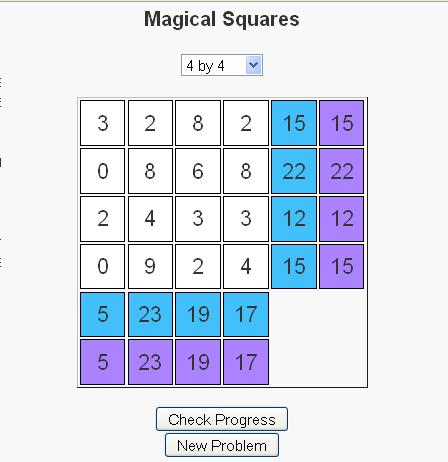# Magical Squares Game

Whether introduced as children in elementary school, as adults in the workplace, or somewhere in between, the concept of magic squares has fascinated people for centuries; The Wikipedia article has discoveries of magic squares dating back to 650 B.C. in China.

Magic Squares of size n (for n >= 3) are n by n grids where the numbers 1, 2, …, n2 are specially arranged such that the sum of each row, column, and diagonal all sum to the same number. Below are two examples of magic squares of size 3 and 4.

 8 3 4 1 5 9 6 2 7
 4 1 16 13 15 11 2 6 10 14 7 3 5 8 9 12

Notice first, that in the first square all the numbers 1, 2, 3, .., 9 are used in the square. Likewise, the numbers 1, 2, …, 15, 16 are used in the second square. Second notice that each row, column and diagonal sums to 15 in the first square and 34 in the second square.

I have recently published a puzzle that is based on the concept of magic squares. There are some slight differences though.
– First, instead of using the numbers 1, 2, …, n2 a random set of numbers are generated.
– Second, the rows and columns each have a desired sum that we would like the numbers in the row/column to sum to.These two changes allow for a puzzle concept to be formulated based on the magic square concept. Users take turns swapping elements until the numbers in each row and column sum to the number in their goal cell, which is located in the last column or row of the grid. Above is an image of a solved puzzle.

Users can determine their progress the numbers in the next-to-last column, which tells the current sum of the numbers in that respective row or column.

To swap two numbers, first click on a cell with one of the two numbers in it. The cell should then turn red. Then click on the cell with the other number in it and the numbers should swap. If you click on the same cell twice no action should take place (except for the cell to turn red and then blank again).

Take a moment to check out the puzzles and let me know what you think.

### Similar Posts:

This site uses Akismet to reduce spam. Learn how your comment data is processed.